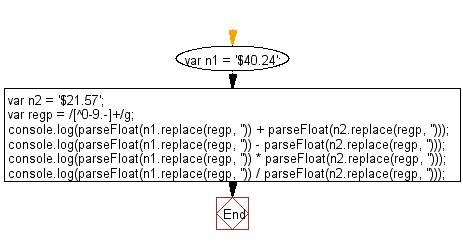# JavaScript: Currency math (add, subtract, multiply, division etc.)

## JavaScript Math: Exercise-25 with Solution

Write a JavaScript function to do currency math (add, subtract, multiply, divide etc.).

Test Data :
n1 = '\$40.24', n2 = '\$21.57';

Sample Solution:-

HTML Code:

``````<!DOCTYPE html>
<html>
<meta charset="utf-8">
<title>Write a JavaScript function to make currency math (add, subtract, multiply, division etc.).</title>
<body>

</body>
</html>
```
```

JavaScript Code:

``````var n1 = '\$40.24';
var n2 = '\$21.57';
var regp = /[^0-9.-]+/g;

console.log(parseFloat(n1.replace(regp, '')) +       parseFloat(n2.replace(regp, '')));

console.log(parseFloat(n1.replace(regp, '')) -         parseFloat(n2.replace(regp, '')));

console.log(parseFloat(n1.replace(regp, '')) *         parseFloat(n2.replace(regp, '')));

console.log(parseFloat(n1.replace(regp, '')) /         parseFloat(n2.replace(regp, '')));
```
```

Sample Output:

```61.81
18.67
867.9768
1.865554010199351
```

Pictorial Presentation:Flowchart:Live Demo:

See the Pen javascript-math-exercise-25 by w3resource (@w3resource) on CodePen.

Improve this sample solution and post your code through Disqus

What is the difficulty level of this exercise?

Test your Programming skills with w3resource's quiz.

﻿